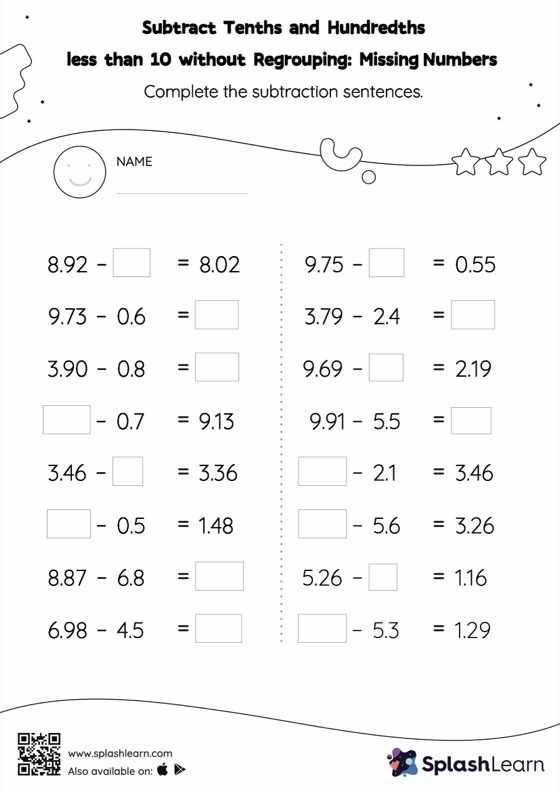# Subtract Tenths and Hundredths less than 10 without Regrouping: Missing Numbers Worksheet

Home > Subtract Tenths and Hundredths less than 10 without Regrouping: Missing NumbersStudents demonstrate their problem-solving ability as they work on this subtract tenths and hundredths less than 10 without regrouping worksheet. Students find the missing number while subtracting decimals by using the relationship between addition and subtraction. Subtract tenths and hundredths less than 10 without regrouping worksheet does not require them to regroup numbers. In each problem, the numbers are laid out in the horizontal format. Students should try to use different strategies involving composing and decomposing numbers to solve these problems. This will help them develop flexibility and fluency.